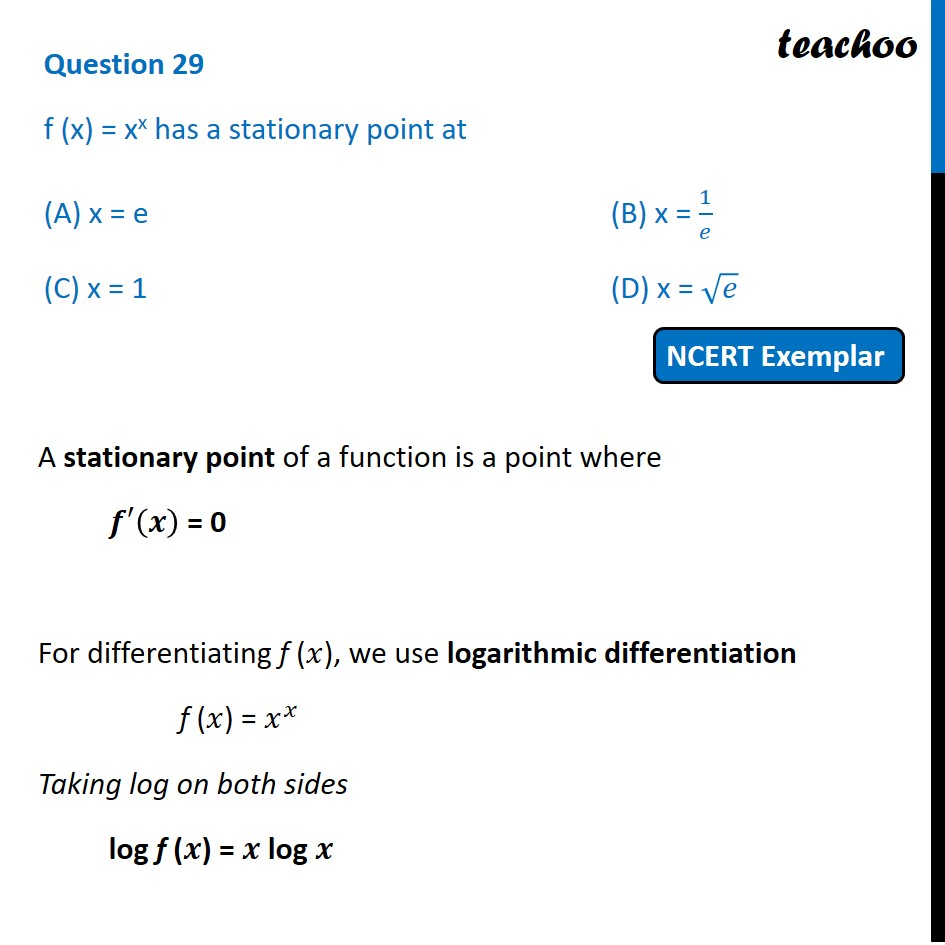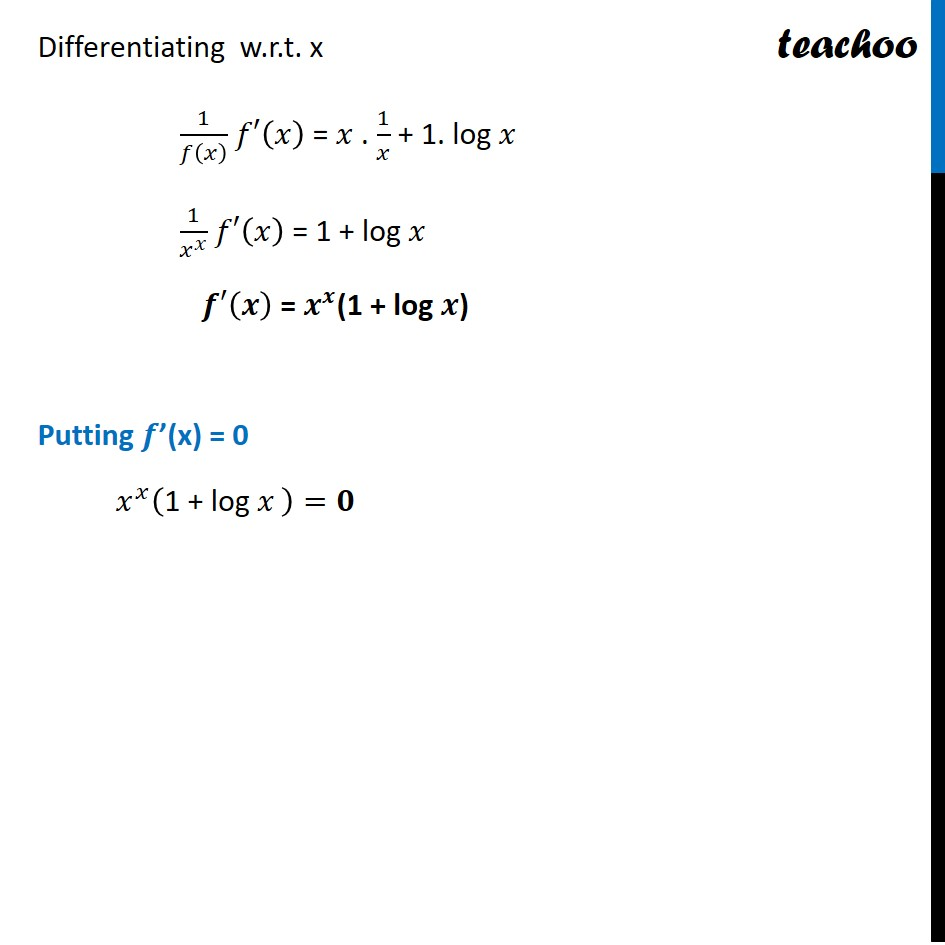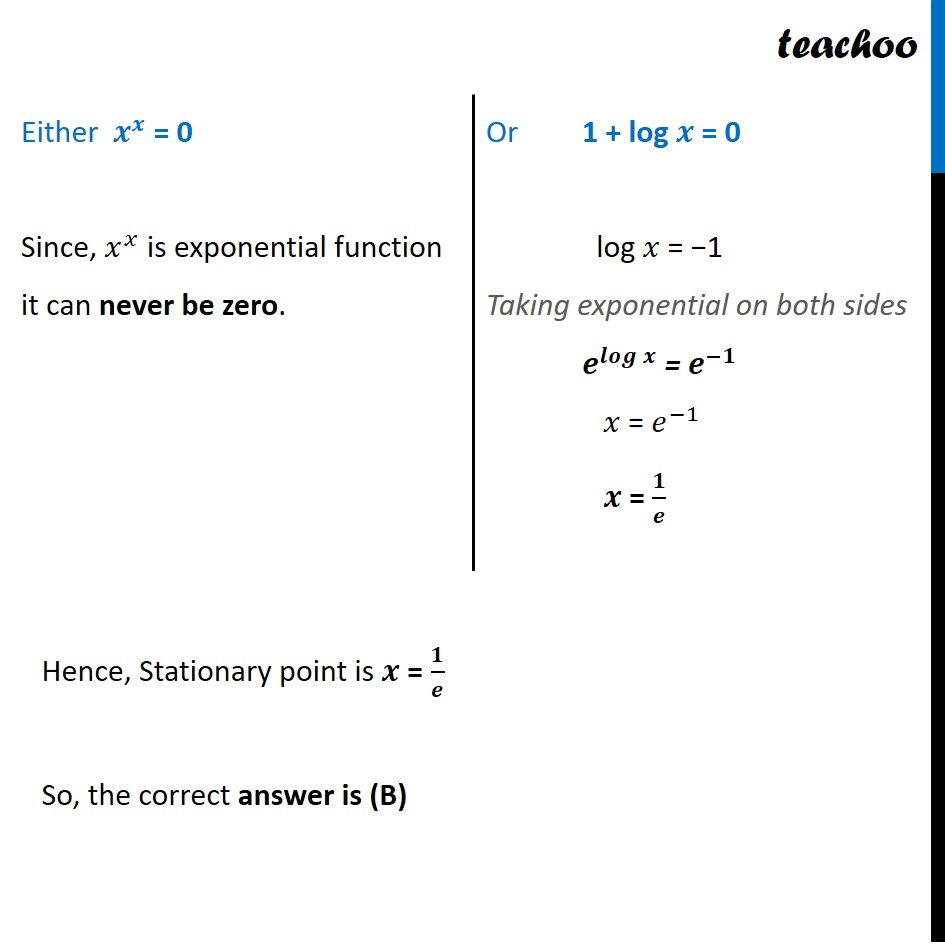NCERT Exemplar - MCQs

Chapter 6 Class 12 Application of Derivatives
Serial order wise

## (C) x = 1                      (D) x = √eGet live Maths 1-on-1 Classs - Class 6 to 12

### Transcript

Question 29 f (x) = xx has a stationary point at (A) x = e (B) x = 1/𝑒 (C) x = 1 (D) x = √𝑒 A stationary point of a function is a point where 𝒇′(𝒙) = 0 For differentiating f (𝑥), we use logarithmic differentiation f (𝑥) = 𝑥^𝑥 Taking log on both sides log f (𝒙) = 𝒙 log 𝒙 Differentiating w.r.t. x 1/𝑓(𝑥) 𝑓′(𝑥) = 𝑥 . 1/𝑥 + 1. log 𝑥 1/𝑥^𝑥 𝑓′(𝑥) = 1 + log 𝑥 𝒇′(𝒙) = 𝒙^𝒙(1 + log 𝒙) Putting 𝒇’(x) = 0 𝑥^𝑥 ("1 + log " 𝑥" " )=𝟎 Either 𝒙^𝒙 = 0 Since, 𝑥^𝑥 is exponential function it can never be zero. Or 1 + log 𝒙 = 0 log 𝑥 = −1 Taking exponential on both sides 𝒆^𝒍𝒐𝒈⁡𝒙 = 𝒆^(−𝟏) 𝑥 = 𝑒^(−1) 𝒙 = 𝟏/𝒆 Hence, Stationary point is 𝒙 = 𝟏/𝒆 So, the correct answer is (B)